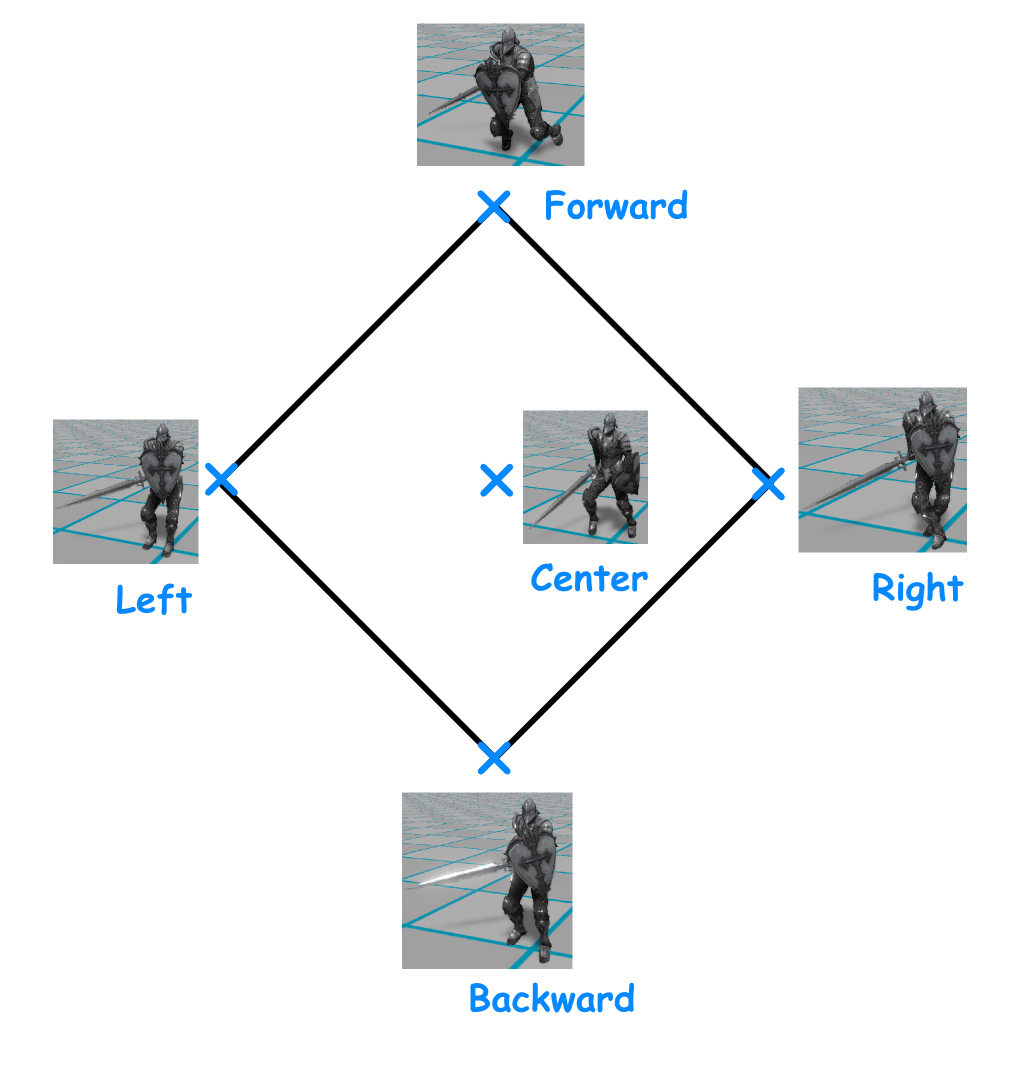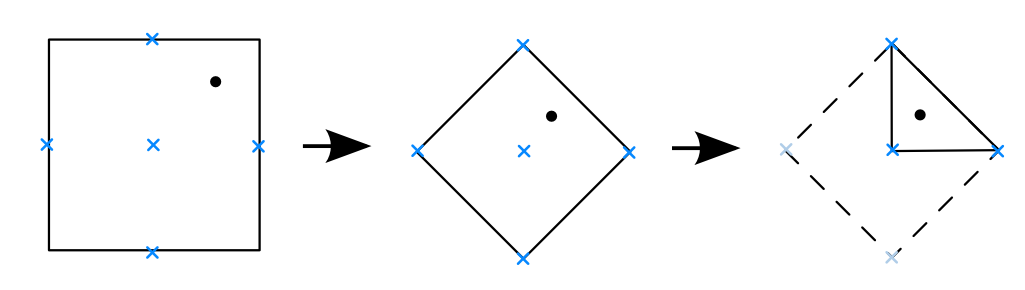### Transforming points in unit square with p-norm

Introduction
Continue with previous post talking about some little tricks I used when implementing the animation editor. This time, I will talk about another maths problem when implementing the directional blend node in the animation tree editor. In this node, it blends 5 different animations(i.e. forward, backward, left, right and center) according to the current direction:blending 5 different animation according to the current direction

And the UI of this node has a square input region like this for artist to preview how those animations are blended together:

So, to compute a blended output, we need to transform the points in the square region to a rhombus, and then we can use barycentric coordinates to determine the blend weight for blending:Transform the current direction for computing the blend weight

p-norm
After searching google for a while, something called Lp-space has shown up with the following figures which looks similar to what I need:Showing "unit circle" with different p-norm

All of the above figures are showing a "unit circle" (i.e. the vectors are of unit-length), but measured with different p-norms. The definition of p-norm is:

This equation describe the length of a vector. When p=2, it is the Euclidean norm that we normally use. Our goal is to transform points inside the unit square to the rhombus, in other words, we want the transformed points having the same length as before the transformation but measured with different p-norm, using p=1 after the transformation and p=infinity before transformation. In other words, we have (assume only dealing with the 2D case, and only consider points in the first quadrant):

, where v=(x, y) is the original point and v'=(x', y') is the transformed point.

Mapping the points
As our target is to find a function to transform the points, having only the above equation is not enough as there are infinitely many way to transform the point that satisfy the above equation. So we need another equation to find a unique solution, the idea is to have the transformed point, original point and the origin are lying on the same straight line(i.e. having the same slope), which gives another equation:
By solving the above equations, which gives the transform functions:
Taking into account those points in other quadrant and special case, which gives the final mapping functions:

However, taking the limit p to infinity and compute it with Wolfram Alpha does not gives any useful result... So I just take p=10 (taking a too large value will result in floating point precision problem...). Here is the plot of how the points are transformed/squeezed into the rhombus:Graph showing how points are transformed from square to rhombus

Although the function cannot transformed the points into a perfect rhombus, it does not have too much artifact when used for animation blending.

Conclusion
In this post, I have described how to map points inside a unit square to a "rhombus" which used for animation blending. But the problem is not completely solved as I am not taking the limit of p to infinity, and there are some power function in the transformation which is not fast... But this is enough for calculating the blend weight of the animations.

Reference
 Lp space: http://en.wikipedia.org/wiki/Lp_space

#### 1 則留言:

1.